• +91 9971497814
• info@interviewmaterial.com

# Chapter 11 Conic Sections Ex-11.1 Interview Questions Answers

### Related Subjects

Question 1 : Find the equation of the circle with centre (0, 2) and radius 2

The equation of a circle with centre (hk)and radius is given as

(x­ – h)2 + (y ­– k)2 = r2

It is given that centre (hk)= (0, 2) and radius (r) = 2.

Therefore, the equation ofthe circle is

(x – 0)2 + (y –2)2 = 22

x2 + y2 + 4 ­– 4 y =4

x2 + y2 ­– 4y =0

Question 2 : Find the equation of the circle with centre (–2, 3) and radius 4

The equation of a circle with centre (hk)and radius is given as

(x­ – h)2 + (y ­– k)2 = r2

It is given that centre (hk)= (–2, 3) and radius (r) = 4.

Therefore, the equation ofthe circle is

(x + 2)2 + (y –3)2 = (4)2

x2 + 4x + 4+ y2 – 6y + 9 = 16

x2 + y2 + 4– 6y –3 = 0

Question 3 : Find the equation of the circle with centre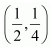and radius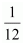The equation of a circle with centre (hk)and radius is given as

(x­ – h)2 + (y ­– k)2 = r2

It is given that centre (hk)=and radius (r) =.

Therefore, the equation ofthe circle is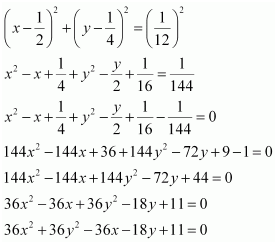Question 4 : Find the equation of the circle with centre (1, 1) and radius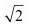The equation of a circle with centre (hk)and radius is given as

(x­ – h)2 + (y ­– k)2 = r2

It is given that centre (hk)= (1, 1) and radius (r) =.

Therefore, the equation ofthe circle is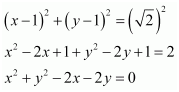Question 5 : Find the equation of the circle with centre (–a, –b) and radius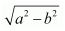The equation of a circle with centre (hk)and radius is given as

(x­ – h)2 + (y ­– k)2 = r2

It is given that centre (hk)= (–a, –b) and radius (r) =.

Therefore, the equation ofthe circle is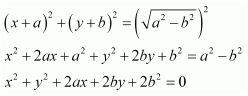Question 6 :

Find the centre and radius of the circle (x +5)2 + (y – 3)2 = 36

The equation of the given circle is (x +5)2 + (y – 3)2 = 36.

(x + 5)2 + (y –3)2 = 36

{x – (–5)}2 + (y –3)2 = 62, which is of the form (x – h)2 + (y – k)2 = r2, where h =–5, k = 3, and r = 6.

Thus,the centre of the given circle is (–5, 3), while its radius is 6.

Question 7 :

Find the centre and radius of thecircle x2 + y2 – 4x – 8y –45 = 0

The equation of the given circle is x2 + y2 – 4x – 8y –45 = 0.

x2 + y2 – 4x – 8y –45 = 0

(x2 – 4x) + (y– 8y) = 45

{x2 – 2(x)(2) + 22} + {y2 – 2(y)(4)+ 42} – 4 –16 = 45

(x – 2)2 + (y –4)2 = 65

(x – 2)2 + (y –4)2 =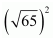, which is of the form (x – h)2 + (y – k)2 = r2, where h =2, k = 4, and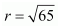.

Thus,the centre of the given circle is (2, 4), while its radius is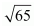.

Question 8 :

Find the centre and radius of thecircle x2 + y2 – 8x +10y – 12 = 0

The equation of the given circle is x2 + y2 – 8x +10y – 12 = 0.

x2 + y2 – 8x +10y – 12 = 0

(x2 – 8x) + (y+ 10y) = 12

{x2 – 2(x)(4) + 42} + {y+ 2(y)(5) + 52}– 16 – 25 = 12

(x – 4)2 + (y +5)2 = 53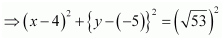which is of the form (x – h)2 + (y – k)2 = r2, where h =4, k = –5, and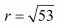.

Thus,the centre of the given circle is (4, –5), while its radius is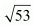.

Question 9 :

Find the centre and radius of the circle 2x2 + 2y2 – x =0

The equation of the given circle is 2x2 + 2y2 – x =0.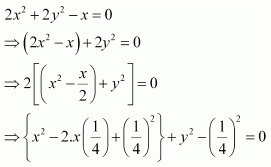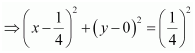, which is of the form (x – h)2 + (y – k)2 = r2, where h =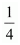k = 0, and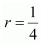.

Thus,the centre of the given circle is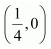, while its radius is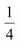.

Question 10 : Find the equation of the circle passing through the points (4, 1) and (6, 5) and whose centre is on the line 4x + y = 16.

Let the equation of the required circle be (x – h)2 + (y – k)2 = r2.

Since the circle passesthrough points (4, 1) and (6, 5),

(4 – h)2 + (1 – k)2 = r2 … (1)

(6 – h)2 + (5 – k)2 = r2 … (2)

Since the centre (h, k) of the circlelies on line 4x + y = 16,

4h + k = 16 …(3)

From equations (1) and(2), we obtain

(4 – h)2 + (1 – k)= (6 – h)2 + (5 – k)2

16 – 8h + h2 + 1 – 2k + k2 = 36 – 12h + h2 + 25 – 10k + k2

16 – 8h + 1 –2k = 36 – 12h + 25 – 10k

4h + 8k =44

h + 2k =11 … (4)

On solving equations (3) and (4), weobtain h = 3 and k = 4.

On substituting the values of h and k inequation (1), we obtain

(4 – 3)2 + (1 – 4)2 = r2

(1)2 + (– 3)2 = r2

1 + 9 = r2

r2 = 10

Let the equation of the required circle be (x – h)2 + (y – k)2 = r2.

Since the circle passesthrough points (4, 1) and (6, 5),

(4 – h)2 + (1 – k)2 = r2 … (1)

(6 – h)2 + (5 – k)2 = r2 … (2)

Since the centre (h, k) of the circlelies on line 4x + y = 16,

4h + k = 16 …(3)

From equations (1) and(2), we obtain

(4 – h)2 + (1 – k)= (6 – h)2 + (5 – k)2

16 – 8h + h2 + 1 – 2k + k2 = 36 – 12h + h2 + 25 – 10k + k2

16 – 8h + 1 –2k = 36 – 12h + 25 – 10k

4h + 8k =44

h + 2k =11 … (4)

On solving equations (3) and (4), weobtain h = 3 and k = 4.

On substituting the values of h and k inequation (1), we obtain

(4 – 3)2 + (1 – 4)2 = r2

(1)2 + (– 3)2 = r2

1 + 9 = r2

r2 = 10

Thus, the equation of therequired circle is

(x – 3)2 + (y –4)2 =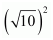x2 – 6x + 9+ y2 ­– 8y + 16 = 10

x2 + y2 – 6x – 8y +15 = 0

Todays Deals### Chapter 11 Conic Sections Ex-11.1 Contributorskrishan

Name:
Email:

# Latest News# 9000 interview questions in different categories